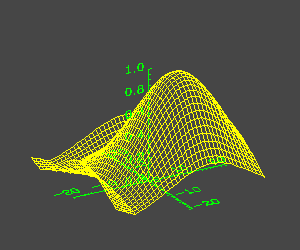# Drawing Surface Axes Through the Origin

QUESTION: I want to use the SURFACE command to render the surface of my data, but I don't like where IDL places the axes. I would prefer the axes go through the origin, which is more or less in the center of my data. Can IDL do this?ANSWER: Yes, you can use the AXIS command to draw a plot axis in any location on the plot. The trick here is to know how to turn the normal surface axes off and how to use the SURFACE command to create and save the 3D transformation matrix that you will use with the AXIS command to position the new axes correctly. Here is an example of how to do it.

First, create some data for surface display. You can use the DIST and SHIFT commands like this:

```   peak = SHIFT(DIST(40), 40./2+5, 40./2-5)
peak = EXP(-(peak/15.)^2)
x = FINDGEN(40) - 20.0
y = FINDGEN(40) - 20.0
```

Next, load a few drawing colors for displaying the data.

```   TVLCT, [255, 0, 70], [255, 255, 70], [0, 0, 70], 1
```

Now you are ready to set up the 3D transformation matrix. You will save it in the !P.T system variable by setting the SAVE keyword. The normal axis display and positioning is turned off with the [XYZ]STYLE keywords. The background of the plot is drawn in a charcoal color. Your command looks like this:

```   SURFACE, peak, x, y, /NoData, BackGround=3, \$
XStyle=4, YStyle=4, ZStyle=4, /Save
```

Next, you are ready to draw the axes with the AXIS command. Each axis will be drawn through the origin with a green color. Notice the T3D keyword, which indicates that the transformation matrix in the !P.T system variable should be used to position the axis. Your code looks like this:

```   AXIS, /XAxis, 0, 0, 0, /T3D, CharSize=1.5, Color=2
AXIS, /YAxis, 0, 0, 0, /T3D, CharSize=1.5, Color=2
AXIS, /ZAxis, 0, 0, 0, /T3D, CharSize=1.5, Color=2
```

Finally, you are ready to draw the surface on the new axes, like this:

```   SURFACE, peak, x, y, /NoErase, Color=1, \$
XStyle=4, YStyle=4, ZStyle=4

```Here is an example of surface of the data above drawn with the axes located at the origin.[Return to IDL Programming Tips]